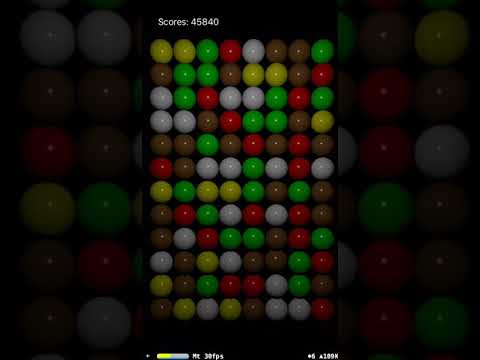/ Games

# Library for simple Match3 games## Match3Kit

Library for simple Match3 games. It can work with a solid grid of figures and spill them only in columns.

## Example

Create the enum for all kinds of figures:

``````typealias MyGrid = Grid<Figure>
typealias MyController = Controller<Figure>

enum Shapes: String, GridFilling {
case square
case circle
case triangle

var pattern: Pattern {
Pattern(indices: [])
}
}
``````

Create a grid controller with configurations:

``````let controller = MyController(size: Size(columns: 6, rows: 6),
basic: [.square, .circle, .triangle],
bonuse: [],
generatorType: Generator<Toys>.self,
matcherType: Matcher<Toys>.self)
``````

Create UI based on the grid that the controller generated:

``````for index in allIndices {
let cell = controller.grid.cell(at: index)
setupUI(for: cell, at: index)
}
``````

Swap figures after the user interaction:

``````func swap(source: Index, target: Index) {
if controller.canSwapCell(at: source, with: target) {
swapUI(source, target)
if controller.shouldSwapCell(at: source, with: target) {
let indices = controller.swapAndMatchCell(at: source, with: target)
let match = controller.match(indices: indices, swapIndices: [source, target])
remove(indices)
spawn(match.spawned)
spill(match.removed)
} else {
swapUI(source, target)
}
}
}
``````